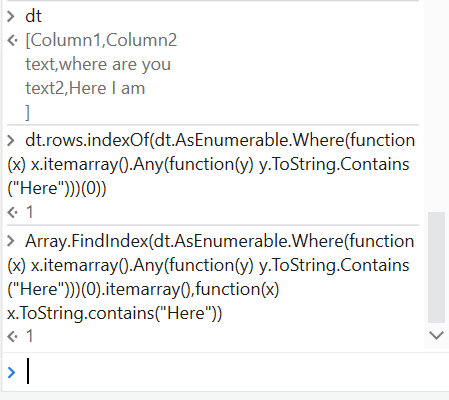# Search Complete Data table and get first Column Data

Hi Everyone

I have a Data table consisting of 20+ columns and 5000+ rows.

I like to avoid using a for each loop as it will be time consuming so is there an efficient way of searching the WHOLE data table to see if it contains the variable “Unique” and get the data of that row and first column where its found.

Thanks

Hi @CGhoST,

Sharing my suggestion on the topic you posted.

We could utilise the lookup data table activity to avoid for each row loop.

But lookup data table activity only search for the given column name or index to search the given value.

For work around we can get the column number count from the data table or excel.

Loop the column index this will loop only max 20 times and enter this column index in the lookup data table to improve the search in all the columns to get the cell reference.

It might be other work around. Please try to do the above approach and let us know. For reference please review the below link.

https://docs.uipath.com/activities/docs/lookup-data-table

@CGhoST

`Rowindex = dt.rows.indexof(dt.AsEnumerable.Where(function(x) x.itemarray().contains("yourvariable or text"))(0))`

`Columnindex = Array.IndexOf(dt.AsEnumerable.Where(function(x) x.itemarray().contains("yourvariable or text"))(0).itemarray(),"your variable or text")`

Hope this helps

Cheers

@kirankumar.mahanthi1

That was an option i was considering but there are many files it needs to iterate through so was looking for one line assign activity.

@CGhoST

Always going for linq expression are good and to avoid looping. Try with @Anil_G suggestion. Thanks for your response.

@Anil_G

I tried Rowindex and all results are coming with “-1”

When i tried Columnindex i got “Assign: Object reference not set to an instance of an object.”

Thanks

@CGhoST

`rowindex = dt.rows.indexOf(dt.AsEnumerable.Where(function(x) x.itemarray().Any(function(y) y.ToString.Contains("Text Here")))(0))`

`columnindex = Array.FindIndex(dt.AsEnumerable.Where(function(x) x.itemarray().Any(function(y) y.ToString.Contains("Text Here")))(0).itemarray(),function(x) x.ToString.contains("Text Here"))`

Or

you can use like this as well directly as you got the row index already

`columnindex = Array.FindIndex(dt.Rows(rowindex).itemarray(),function(x) x.ToString.contains("Text Here"))`Hope this helps

cheers

It works,

Thank you so much. I would never have figured this out.

1 Like

@CGhoST

Happy Automation

From next time I am sure you will use them elsewhere

Cheers

This topic was automatically closed 3 days after the last reply. New replies are no longer allowed.